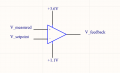# Comparator with linear output to equalize inputs

#### MarcWeissfloch

Joined Oct 20, 2022
2
Hello!
I am a ME dabbling in some EE projects so forgive me if this sounds clueless ! I am developing a current feedback system for a power supply.

I am looking for an analog device that will adjust the output to keep the difference between the inputs at zero.
In other words, if V_measured is lower that V_setpoint the output will go up in a linear fashion until the inputs equalize or it reaches +3.6V.
If V_measured is higher than V setpoint the output will go down in a linear fashion until the inputs equalize or it reaches +1.1V.

Does such a device exist? Or is there a simple way of implementing this using analog parts?

Thanks, Marc#### WBahn

Joined Mar 31, 2012
28,159
It's not enough to say that the output response is linear, you need a gain. At what difference between V_measured and V_setpoint do you want the output to transition from the linear region to the saturated region in both directions.

When you say that you want it to go up until the inputs equalize, well, if they equalize then the difference is zero and your output would be zero (if you want the output to have a linear relationship to the difference of the inputs).

So think carefully about whether this is really what you want.

What you are heading down the road to making right now is a "proportional controller", whose output requires a non-zero error signal in order to produce a non-zero control signal.

If you want the controller to maintain a non-zero output even after the error has been reduced to zero, then you need a "proportional-integral controller", which will eliminate steady-state error, but introduces instability. If the instability is too much, you can add yet another component and make a "proportional-integral-derivative controller" to get the stability back.

#### LowQCab

Joined Nov 6, 2012
2,874
If You would give a comprehensive statement outlining
the problem that You are trying to solve,
You would probably get more appropriate answers.
.
.
.

#### MarcWeissfloch

Joined Oct 20, 2022
2
WBahn: Thanks for your reply! Yes I am needing an output that is non zero and not propotional to a fixed voltage which is why I can't simply use an OpAmp. I probably shouldn't have used an opamp to illustrate, sorry!

My system will yield a V_measured that will equal V_setpoint when V_feedback is somewhere between 3.3 and 1.1V. There is already a feedback system that is keeping everything stable. What I need now is an adjustment that will slowly drift the output until the 2 inputs are equal.

#### WBahn

Joined Mar 31, 2012
28,159
WBahn: Thanks for your reply! Yes I am needing an output that is non zero and not propotional to a fixed voltage which is why I can't simply use an OpAmp. I probably shouldn't have used an opamp to illustrate, sorry!

My system will yield a V_measured that will equal V_setpoint when V_feedback is somewhere between 3.3 and 1.1V. There is already a feedback system that is keeping everything stable. What I need now is an adjustment that will slowly drift the output until the 2 inputs are equal.
I'm having to read between the lines here and there are a couple of (possibly wrong) conclusions I am inferring.

When you say "until the 2 inputs are equal", how "equal" do they have to be in order to be "equal enough"? If V_measured is 2.31 V and V_setpoint is 2.33 V, is that too unequal, or is that equal enough? If that's not equal enough, what about if V_measured is 2.36152 V and V_setpoint is 2.36149 V, is that now equal enough, or does it need to still be better?

The description of something that is going to slowly drift the output until the two are equal is a pretty fair description of what an integrator does, But you may not need it because you might find that the residual error term on a purely proportional controller is good enough.